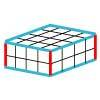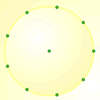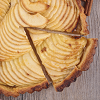#### You may also like### Making Cuboids

Let's say you can only use two different lengths - 2 units and 4 units. Using just these 2 lengths as the edges how many different cuboids can you make?How many DIFFERENT quadrilaterals can be made by joining the dots on the 8-point circle?### Pumpkin Pie Problem

Peter wanted to make two pies for a party. His mother had a recipe for him to use. However, she always made 80 pies at a time. Did Peter have enough ingredients to make two pumpkin pies?

# Round and Round the Circle

##### Age 7 to 11Challenge Level

From Mounts Bay School we had solutions in from Jamie, Luke, Cory, Luke, Joseph, Ben, Kara, Merrion, Katie, Ben, Chris, Ellen, Emma, Liam and Heidi. Here are three of them;

Certain numbers are the same. I think that numbers which are half of another number have double the amount of trails around the circle. This happens because when you try the numbers $8$ and $4$ they are exactly the same.

In the middle of each shape there is a perfect square. We can tell it is a perfect square because the angles are all right angles. We all figured it out together by looking and trying to do a number $8$ when we noticed the squares. We did not think it was a coincidence!

$12$ is equal
$11$ is the same as $1$ except backwards
$10$ is the same as $2$ except backwards
$09$ is the same as $3$ except backwards
$08$ is the same as $4$ except backwards
$07$ is the same as $5$ except backwards
$06$ is just a recurring straight line
All others are in reverse.
The pattern is that as the numbers from $11$ go down, the numbers which they are the same go up.

At the very end of the month we had two comments in from Morgan, Sara, Billie and Lucy from Southam St. James Primary School:

If the number of points are prime, all numbers will work but if the number of points are not prime all numbers apart from factors of the number of points will work.

and

If there's an even number of points odd numbers of steps won't work. If there's an odd number of points even steps won't work. If there's a prime number all of them work.